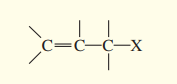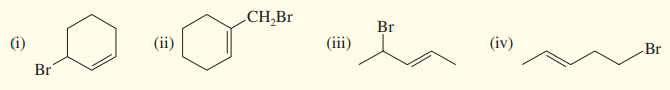×
Get Full Access to Organic Chemistry - 8 Edition - Chapter 6 - Problem 52sp
Get Full Access to Organic Chemistry - 8 Edition - Chapter 6 - Problem 52sp

×

# Allylic halides have the structure (a) Show how theISBN: 9780321768414 33

## Solution for problem 52SP Chapter 6

Organic Chemistry | 8th Edition

• Textbook Solutions
• 2901 Step-by-step solutions solved by professors and subject experts
• Get 24/7 help from StudySoup virtual teaching assistantsOrganic Chemistry | 8th Edition

4 5 1 429 Reviews
20
1
Problem 52SP

Allylic halides have the structure(a) Show how the first-order ionization of an allylic halide leads to a resonance-stabilized cation.

(b) Draw the resonance structures of the allylic cations formed by ionization of the following halides.

(c) Show the products expected from $$S_{N} 1$$ solvolysis of these halides in ethanolEquation Transcription:Text Transcription:

C=C-C-X

S_N1

Br

CH2Br

Br

Br

Step-by-Step Solution:

Solution:

Step 1

The structure of allylic halide is,(a) The  first-order ionization of an allylic halide and the resonance stabilization is given below-

In this 1st order ionization process, the halide group (X), from the allyl halide has been released as X- ion and forms a carbocation. This carbocation has two resonance structures.Step 2 of 3

Step 3 of 3

##### ISBN: 9780321768414

Since the solution to 52SP from 6 chapter was answered, more than 1444 students have viewed the full step-by-step answer. This full solution covers the following key subjects: allylic, halides, resonance, ionization, ethanol. This expansive textbook survival guide covers 25 chapters, and 1336 solutions. This textbook survival guide was created for the textbook: Organic Chemistry, edition: 8. The answer to “?Allylic halides have the structure (a) Show how the first-order ionization of an allylic halide leads to a resonance-stabilized cation.(b) Draw the resonance structures of the allylic cations formed by ionization of the following halides. (c) Show the products expected from $$S_{N} 1$$ solvolysis of these halides in ethanol Equation Transcription:Text Transcription:C=C-C-XS_N1BrCH2BrBrBr” is broken down into a number of easy to follow steps, and 52 words. Organic Chemistry was written by and is associated to the ISBN: 9780321768414. The full step-by-step solution to problem: 52SP from chapter: 6 was answered by , our top Chemistry solution expert on 05/06/17, 06:41PM.

## Discover and learn what students are asking

Statistics: Informed Decisions Using Data : Estimating the Value of a Parameter
?What does the 95% represent in a 95% confidence interval?

Statistics: Informed Decisions Using Data : The Randomized Complete Block Design
?Given the following ANOVA output, answer the questions that follow: (a) The researcher wants to test H0: m1 = m2 = m3 =

Unlock Textbook Solution